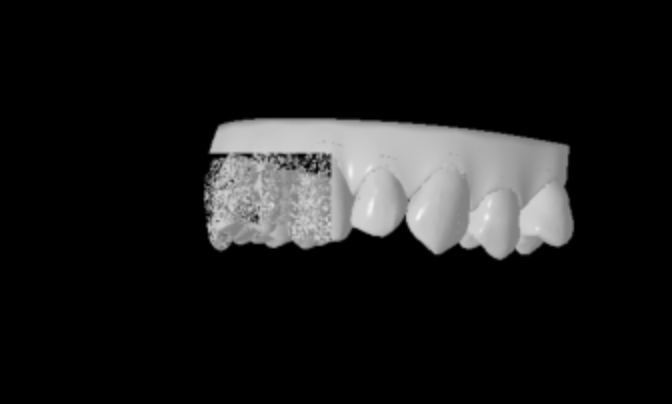Ask questionsRendering of occluded objects looks strange

Hello,

I'm trying to render teeth arch using pytorch3d and got strange result (please see screenshot):When I removed some occluded teeth rendered image was ok. I've tried to decimate mesh and reduced number of faces by 4 times and rendering was ok. Is it some limitation of framework? Could you please help me with this issue?

Here is code to reproduce:

``````import torch
import numpy as np
import matplotlib.pyplot as plt
import trimesh

from pytorch3d.structures import Meshes, Textures
from pytorch3d.transforms import euler_angles_to_matrix

from pytorch3d.renderer import (
OpenGLPerspectiveCameras, RasterizationSettings, MeshRenderer, MeshRasterizer, BlendParams,
)

device = torch.device("cuda:0")
torch.cuda.set_device(device)

stl_file = "teeth.stl"

verts, faces = torch.from_numpy(mesh.vertices).float(), torch.from_numpy(mesh.faces)
verts /= 1000

verts_rgb = torch.ones_like(verts)[None]
textures = Textures(verts_rgb=verts_rgb.to(device))

mesh = Meshes(
verts=[verts.to(device)],
faces=[faces.to(device)],
textures=textures
)

return mesh

cameras = OpenGLPerspectiveCameras(device=device, fov=0.27, degrees=False)
blend_params = BlendParams(sigma=1e-2, gamma=1e-7)
raster_settings = RasterizationSettings(
image_size=1024,
faces_per_pixel=10,
)

lights = PointLights(device=device, location=((2.0, -2.0, -2.0),))
phong_renderer = MeshRenderer(
rasterizer=MeshRasterizer(
cameras=cameras,
raster_settings=raster_settings
),
)

R = euler_angles_to_matrix(torch.tensor(np.radians([90, 40, 0])).float(), convention="XYZ").unsqueeze(0).cuda()
T = torch.tensor([0, 0, 0.5]).float().unsqueeze(0).cuda()

image_ref = phong_renderer(meshes_world=orig_mesh, R=R, T=T).squeeze()

plt.figure(figsize=(10, 10))
plt.imshow(image_ref.cpu().numpy()[:, :, 1], cmap='gray')
``````